Main MRPT website > C++ reference for MRPT 1.5.7
mrpt::poses::SE_traits< 2 > Struct Template Reference

## Detailed Description

### template<> struct mrpt::poses::SE_traits< 2 >

Specialization of SE for 2D poses.

SE_traits

Definition at line 75 of file SE_traits.h.

#include <mrpt/poses/SE_traits.h>

## Public Types

enum  { VECTOR_SIZE = 3 }

typedef mrpt::math::CArrayDouble< VECTOR_SIZEarray_t

typedef mrpt::math::CMatrixFixedNumeric< double, VECTOR_SIZE, VECTOR_SIZEmatrix_VxV_t

typedef CPose2D pose_t

typedef mrpt::math::TPose2D lightweight_pose_t

typedef mrpt::math::TPoint2D point_t

## Static Public Member Functions

static void exp (const array_t &x, CPose2D &P)
Exponential map in SE(2) More...

static void pseudo_exp (const array_t &x, CPose2D &P)
Pseudo-Exponential map in SE(2), in this case identical to exp() More...

static void ln (const CPose2D &P, array_t &x)
Logarithm map in SE(2) More...

static void pseudo_ln (const CPose2D &P, array_t &x)
A pseudo-Logarithm map in SE(2), where the output = [X,Y, Ln(ROT)], that is, the normal SO(2) logarithm is used for the rotation components, but the translation is left unmodified. More...

static void jacobian_dP1DP2inv_depsilon (const CPose2D &P1DP2inv, matrix_VxV_t *df_de1, matrix_VxV_t *df_de2)
Return one or both of the following 3x3 Jacobians, useful in graph-slam problems: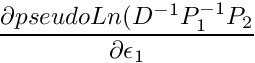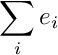With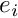and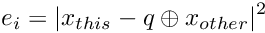being increments in the linearized manifold for P1 and P2. More...

static void jacobian_dDinvP1invP2_depsilon (const CPose2D &Dinv, const CPose2D &P1, const CPose2D &P2, matrix_VxV_t *df_de1, matrix_VxV_t *df_de2)
Return one or both of the following 3x3 Jacobians, useful in graph-slam problems: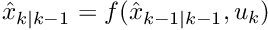Withandbeing increments in the linearized manifold for P1 and P2. More...

## ◆ array_t

 typedef mrpt::math::CArrayDouble mrpt::poses::SE_traits< 2 >::array_t

Definition at line 78 of file SE_traits.h.

## ◆ lightweight_pose_t

 typedef mrpt::math::TPose2D mrpt::poses::SE_traits< 2 >::lightweight_pose_t

Definition at line 81 of file SE_traits.h.

## ◆ matrix_VxV_t

 typedef mrpt::math::CMatrixFixedNumeric mrpt::poses::SE_traits< 2 >::matrix_VxV_t

Definition at line 79 of file SE_traits.h.

## ◆ point_t

 typedef mrpt::math::TPoint2D mrpt::poses::SE_traits< 2 >::point_t

Definition at line 82 of file SE_traits.h.

## ◆ pose_t

 typedef CPose2D mrpt::poses::SE_traits< 2 >::pose_t

Definition at line 80 of file SE_traits.h.

## ◆ anonymous enum

 anonymous enum
Enumerator
VECTOR_SIZE

Definition at line 77 of file SE_traits.h.

## ◆ exp()

 static void mrpt::poses::SE_traits< 2 >::exp ( const array_t & x, CPose2D & P )
inlinestatic

Exponential map in SE(2)

Definition at line 85 of file SE_traits.h.

## ◆ jacobian_dDinvP1invP2_depsilon()

 static void mrpt::poses::SE_traits< 2 >::jacobian_dDinvP1invP2_depsilon ( const CPose2D & Dinv, const CPose2D & P1, const CPose2D & P2, matrix_VxV_t * df_de1, matrix_VxV_t * df_de2 )
static

Return one or both of the following 3x3 Jacobians, useful in graph-slam problems:Withandbeing increments in the linearized manifold for P1 and P2.

## ◆ jacobian_dP1DP2inv_depsilon()

 static void mrpt::poses::SE_traits< 2 >::jacobian_dP1DP2inv_depsilon ( const CPose2D & P1DP2inv, matrix_VxV_t * df_de1, matrix_VxV_t * df_de2 )
static

Return one or both of the following 3x3 Jacobians, useful in graph-slam problems:Withandbeing increments in the linearized manifold for P1 and P2.

## ◆ ln()

 static void mrpt::poses::SE_traits< 2 >::ln ( const CPose2D & P, array_t & x )
inlinestatic

Logarithm map in SE(2)

Definition at line 91 of file SE_traits.h.

## ◆ pseudo_exp()

 static void mrpt::poses::SE_traits< 2 >::pseudo_exp ( const array_t & x, CPose2D & P )
inlinestatic

Pseudo-Exponential map in SE(2), in this case identical to exp()

Definition at line 88 of file SE_traits.h.

## ◆ pseudo_ln()

 static void mrpt::poses::SE_traits< 2 >::pseudo_ln ( const CPose2D & P, array_t & x )
inlinestatic

A pseudo-Logarithm map in SE(2), where the output = [X,Y, Ln(ROT)], that is, the normal SO(2) logarithm is used for the rotation components, but the translation is left unmodified.

Definition at line 96 of file SE_traits.h.

 Page generated by Doxygen 1.8.14 for MRPT 1.5.7 Git: 8277875f6 Mon Jun 11 02:47:32 2018 +0200 at lun oct 28 01:50:49 CET 2019